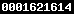dőmúlató.hu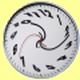collection of free online games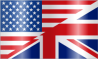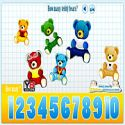Kids counting Teddy bears Kids counting teddy bears there are is a simple game for children who are learning to count.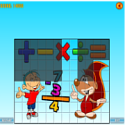PicTrix Math A very special game for kids. Carry the falling picture pieces at exact place to make a full photo.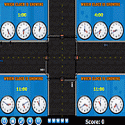Traffic control time This is a game where you control the flow of traffic by starting and stopping the traffic in a lane by clicking a clock which shows the requested time.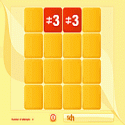Memory math game Let’s test your memory! This time with math signs! Guess the pairs in each level and go to the next one where more squares and more pairs too.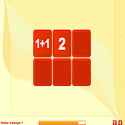Math equations Yet another memory math game, but this time your goal isn't just to test your memory. You've got to resolve math equations.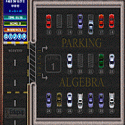Parking algebra This is a mathematics based parking skill game where you need to first solve algebra equation to figure out the right number slot and then park your car in the slot containing that value.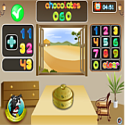Math monster add/sub A puzzle game where you need to solve the addition and subtraction puzzles to close the box of chocolates and scare off the monsters.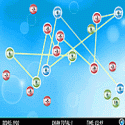Golden zeros Math-skills and strategy based High Score game where you require making a chain of numbered balls to get a sum of zero.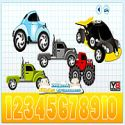Counting hidden cars How many cars there are is a simple car-game where the player learns to count cars and trucks.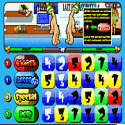Handsome George, 12 year old, is bored. So he uses his imagination to create an adventure for his also imaginary friend HandSome.Hello Kitty math game Test out your math skills by answer 20 questions of this game. At the end of the game, you will be give a grade of your test.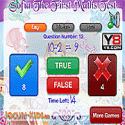Sofia the first maths test Sofia The First back with us with a new game. Sofia The First Maths Test game is a new game with math questions that you must answer in a short time.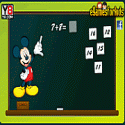Mickey Mouse math game Learning math with Mickey Mouse today.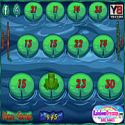Froggy jumps This leggy froggy is no doubt the most enthusiastic little mathematician on the lake!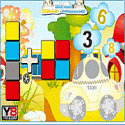Mini taxi car game Help building a mini taxi-car, look for all the necessary pieces in order to build a taxi-car; in the end you will have a superb yellow taxi-car ready to embark in a surreal journey.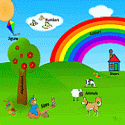Kids workshop It is a game for all kids to advance their learning.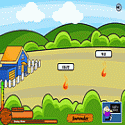Battle math Defend your house from monster attack with your brain.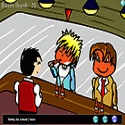The smart drunk Each turn, a player gets to order 1, 2 or 3 more drinks. The goal is to not be the one that drink the 25th glass.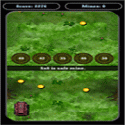Math tank run A math based tank run game. On the way there are several minefield. With the help of math you can escape from these minefields.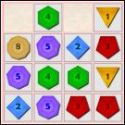17th shape A variation of the 2048 game with deluxe design and 2 power-ups. Slide and combine the tiles with geometrical figures to form more complex ones.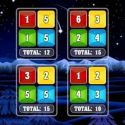Make all equall Move numbers from one box to the other so that the sum of the numbers in each box have a total equal to the level’s target number.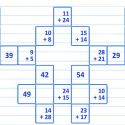Math mahjong A combination of Math and popular chinese game Mahjong. In this game pair would be 2 equal values. Deluxe math test A challenging and entertaining free math game with real time high scoring system.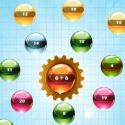Orbiting number In this game you need to remove all the orbiting balls . Just click on the ball which has exactly same value as the ball in the center has.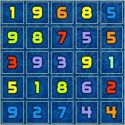Math and match In this game you need to select a number of blocks whose sums equal the given expression.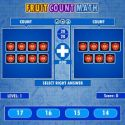Fruit count math In this game you need to count the fruits inside 2 boxes. With the counted numbers of both boxes, you have to solve the asked arithmetic problems.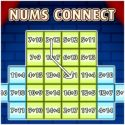Nums connect You have to connect Expressions in each tile at least once to finish a level. Try to make Expressions chains as long as possible to get best score.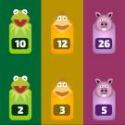Stop invisible In this game there are 4 columns each with a static number. Each time four new number will fall from upside.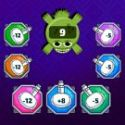Zero logic  is a simple yet challenging math game where you'll try and reduce a Monster's number to zero using math guns.Skyline maker is a simple but addicting puzzle game. In it, you need to add and remove floors to make a building.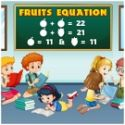Fruits equations  is a math puzzle game. In this game you need to find the values of some fruits by solving given equations. In the game, you will see a board.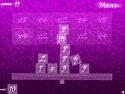Tactic balance Make a percent balance. Collect maximum scores using all of block numbers.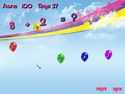Balloon math Throw darts at balloons with the right math answers on them!!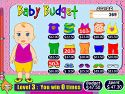Baby budget Buy your baby dresses as much as the budget.